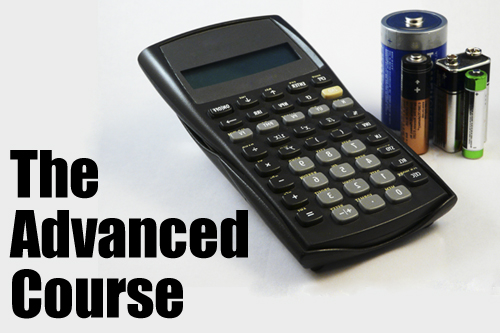Loading... Please wait...

## Popular BrandsHow to Avoid Getting Ripped Off on Battery Lighting – Advanced Course

In our previous article, 9 Ways to Avoid Getting Ripped Off on Battery Lighting, we discussed some tips for spotting disappointing LED lighting products before you get them home. This time around we wanted to equip you with a more comprehensive set of tools, specifically some down and dirty math that will let you evaluate products like an electrical engineer. In fact, the process described below is nearly identical to one we go through when first developing a new product. As with the previous article, this is geared toward products using white LEDs and alkaline batteries.

The Equation: while most consumers probably haven’t dealt with physics equations since high school, nearly every shopper has done a quick dollars divided by ounces calculation in the grocery store to see if the bulk size is really the best buy. The equation we’re going to be using is the same general idea: capacity divided by current equals hours of battery life. In other words, battery capacity (measured in milliamp hours or mAh) divided by the current drawn by the LEDs (measured in milliamps or mA) equals the number of hours the lights will operate. If you’re already starting to feel a little dizzy, don’t worry, it will make sense as we go on.

The Batteries: So we have our basic equation, hours=capacity/current, and now we need to start filling in the pieces. The first thing to know is how much capacity is in a battery. Here we’ve provided a table with the average capacity of the most common alkaline battery sizes. Now you might be tempted to think you should multiply the capacity of the battery type by the number of batteries in the product to get the total capacity. In fact most battery products are wired in what is called a series circuit. Without going into too much detail, this means that each battery increases the product’s voltage, but the overall capacity remains the same. So regardless of the number of batteries in the product, simply take the capacity of the battery type, and you’ve just filled in the capacity component of the equation.

 Battery Capacity (mAh) AAA 1200 AA 2700 C 7800 D 18000

The LEDs: Second component of the equation is current. To achieve optimum brightness the classic plastic dome type white LED we’re all so familiar with draws 20mA. This can get more complicated if the product is using a different kind of LED, but these are one of the most commonly used LEDs types, so to keep things simple we’ll stick with 20mA. The most common arrangement is to connect the LED’s in a parallel circuit (the opposite of a series circuit), so in this case we do multiply the number of LEDs in the product by 20 to get the current.

The Time: Now simply divide the capacity by the current and you have the number of hours the product will operate, assuming the LEDs are running at full brightness. Another thing you can do to test a product’s battery life claim is figure out the capacity and divide it by the number of hours the product claims it will run. If the resulting number is less than 20mA per LED than the product is probably running at less than the maximum brightness of the LEDs.

Example: If things are still a bit fuzzy, let’s go through an example. Below is a table describing a real LED stick-up puck light from a different manufacturer that we purchased and tested. We’ll apply the techniques we’ve just discussed, and see how it holds up.

LED Count: 3

Battery Type: AAA

Batter Life Claim: 100 hours

With AAA batteries at 1200mAh and 3 LEDs at 20mA, we can calculate the expected battery life:

capacity/current=hours: (1200)/( 3*20)= 20 hours

Obviously this doesn’t match the product’s claims, so let’s flip things around. If the light really can operate for 100 hours, how many milliamps are they actually sending to each LED?

capacity/hours=current: (1200)/100 = 12mA which means 4mA for each of the three LEDs

So assuming the battery life claim is accurate, each LED is only going to be putting out 20% of its peak brightness. Depending on your application, such as lighting a dark pantry, this might be an entirely acceptable level of light. But most importantly, if you havean array of options to choose from these simple calculations become a powerful point of comparison between multiple products.